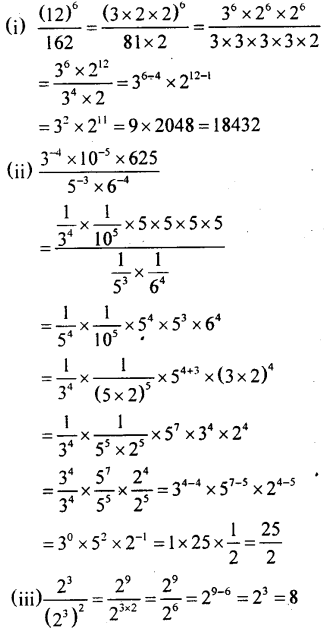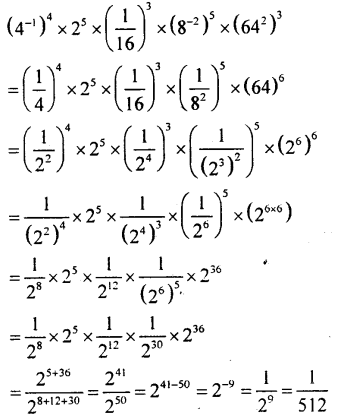# KSEEB Solutions for Class 8 Maths Chapter 10 Exponents Ex 10.7

Students can Download Maths Chapter 10 Exponents Ex 10.7 Questions and Answers, Notes Pdf, KSEEB Solutions for Class 8 Maths helps you to revise the complete Karnataka State Board Syllabus and score more marks in your examinations.

## Karnataka Board Class 8 Maths Chapter 10 Exponents Ex 10.7

Question 1.
Use the laws of exponents and simplifyQuestion 2.
What is the value of $$\frac{\left(10^{3}\right)^{2} \times 10^{-4}}{10^{2}}$$Question 3
Simplify:
$$\left[\frac{b^{-3} \cdot b^{7} \cdot\left(b^{-1}\right)^{2}}{(-b)^{2}\left(b^{2}\right)^{3}}\right]^{-2}$$Question 4.
Find the value of each of the following expressions.

(a). (32)2 – (-2)3)– (-(5)2)2
= 34 – (- 2 x – 2 x – 2)2 – (-52 )2
= 34 – (-23)2 – 54
= 81 – 26 – 625
= 81- 64 – 625
= 81 – 689
= – 608

(b). ((0.6)2)0 – ((4.5)0)-2
= (0.6)2 x 0 – (4.5)0 x -2
= (0.6)0 – (4.5)0
= 1 – 1
= 0

(c).error: Content is protected !!